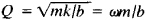# Quality Factor of an Oscillatory System

## Quality Factor of an Oscillatory System

the ratio of the energy stored in an oscillatory system to the energy lost by the system during one oscillation cycle. The quality factor characterizes the grade of the system, since the higher the quality factor of the system, the lower the loss of energy in it during one oscillation.

The quality factor Q of an oscillatory system is associated with the logarithmic decrement 8; at small decrements, Qπ/δ. In an oscillating circuit with inductance L, capacitance C, and ohmic resistance R, the quality factor of the oscillatory system iswhere ω is the natural frequency of the circuit. In a mechanical system with mass m, stiffness k, and friction coefficient b, the quality of an oscillatory system isThe quality factor is a quantitative characteristic of the resonance properties of an oscillatory system, which indicates by what factor the amplitude of stable forced oscillations with resonance exceeds the amplitude of the forced oscillations far from the resonance—that is, in a region of such low frequencies that amplitude of the forced oscillations may be considered independent of frequency. The method for measuring the quality factor of an oscillatory system is based on this property. The degree of quality also characterizes the selectivity of an oscillatory system; the higher the quality factor, the narrower the frequency band of external force that can cause intensive oscillations of the system. Experimentally, the quality factor of an oscillatory system is usually defined as a ratio of the natural vibration frequency to the pass band of the system; that is, Q = ωω. Numerical values of the quality factor of an oscillatory system are 30-100 for a radio-frequency oscillatory circuit; 10,000 for a tuning fork; 100,000 for a piezoelectric quartz plate; and 100-100,000 for a superhigh-frequency cavity resonator.

### REFERENCES

Strelkov, S. P. Vvedenie v teoriiu kolebanii, 2nd ed. Moscow, 1964.
Gorelik, G. S. Kolebaniia i volny, 2nd ed. Moscow, 1959.

V. N. PARYGIN

Site: Follow: Share:
Open / Close